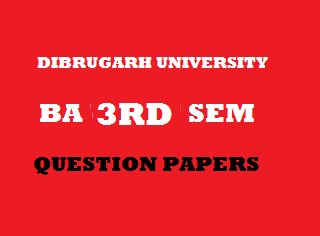## Thursday, January 03, 20192015
(November)
ECONOMICS
(General)
Course: 301
(Public Economics)
Full Marks: 80
Pass Marks: 32 (Backlog)/24 (2014 onwards)
Time: 3 hours
The figures in the margin indicate full marks for the questions

1. Choose the correct option/Answer the following: 1x8=8
1. According to Dr. Dalton, Maximum Social Advantage = ____.
1. MSS > MSB.
2. MSS < MSB.
3. ASB = ASS.
4. MSS = MSB.
1. State one difference between direct and indirect tax.
2. If ed=1, es=1, then the burden of tax will be upon ____.
2. Sellers.
1. According to Dr. Colin Clark, the safe upper limit of taxation is ____ percent of national production.
1. 30.
2. 25.
3. 20.
4. 15.
1. Mention two reasons for the growth of public expenditure in developing countries.
2. Mention two sources of internal public borrowing.
3. Mention one purpose of government budget.
4. The exemption limit of personal income tax for citizens below the age of 60 years, as per the last Union Budget, 2015 – 16 of India is ____.
1. 2 lakhs.
2. 2.5 lakhs.
3. 3 lakhs.
4. 3.5 lakhs.
2. Write short notes on any four of the following (within 150 words each): 4x4=16
1. Public finance and developed economy.
2. Bastable’s cannons of taxation.
3. Public good and merit good.
4. Fiscal policy in attaining price stability.
5. Growth of public debt in India.
6. Main principles of division of financial resources in India.
Answer the following questions (within 500 words each):
3. (a) What is public finance? Explain the subject matter of the study of public finance. 2+9=11
Or
(b) Examine the role of public finance in a developing economy with special reference to India. 11
4. (a) What is tax? Explain the objectives of taxation. 11
Or
(b) Distinguish between absolute and relative taxable capacity. Discuss the factors which determine the taxable capacity of a country. 4+7=11
5. (a) Discuss the impact of public expenditure on production and distribution of income. 6+6=12
Or
(b) Explain the various methods of repayment of public debt. 12
6. (a) Describe the main elements of government budget. Define revenue deficit, fiscal deficit and primary deficit.        7+4=11
Or
(b) Discuss the role of fiscal policy in developing economy. 11
7. (a) Explain the defects of Indian tax system. Mention the steps adapted by Government of India to overcome these defects. 8+3=11
Or
(b) Write a note on the recommendations of the latest Finance Commission of India. 11

***